# 简述【聚类算法】

2016年10月20日 | By News | Filed in: 未分类.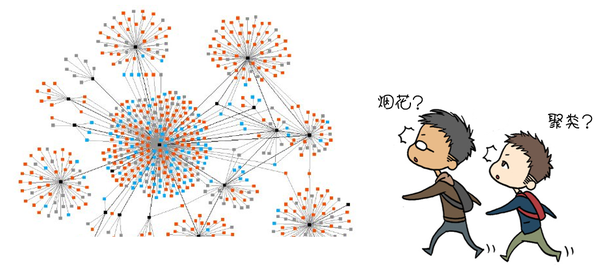所谓人以类聚，物以群分。人都喜欢跟自己像的人聚在一起，这些人或者样子长得比较像，或者身高比较像，或者性格比较像，或者有共同的爱好，也就是身上有某些特征是相似的。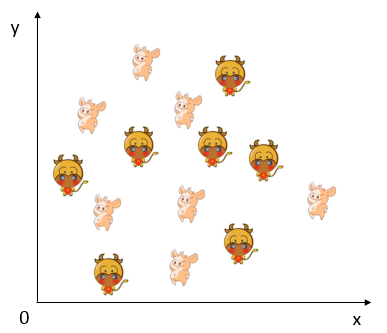前面讲到，聚类算法是根据样本之间的距离来将他们归为一类的，这个距离不是普通的距离，理论上叫做欧氏距离那么聚类算法，是怎么通过迭代的方式，将样本聚成几个类别的呢？

1、在样本中随机选择K个点，作为每个类别的初始中心点，这K是自己定的，假如你想将样本分成3个类K就等于3,4个类K就等于4；

2、计算所有样本离这K个初始中心点的距离并分别进行比较，选出其中最近的距离并把这个样本归到这个初始中心点的类别里，即总共划分成K个类别；

3、舍弃原来的初始中心点，在划分好的K个类别里分别计算出新的中心点，使得这些中心点距离他类别里的所有样本的距离之和最小；

4、判断新获得的中心点是否与旧中心点一样，如不一样则回到第2步，重新计算所有样本离这K个新的中心点的距离并进行比较，选出其中最近的距离并归到这个新的中心点的类别里，继续下面的步奏；如一样则完成，即收敛。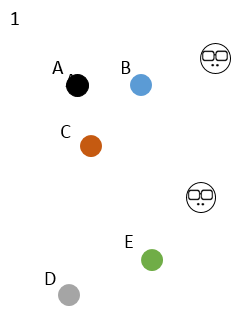有ABCDE5个样本，一开始选定右边的2个初始中心点，K=2，大家颜色都不一样，谁都不服谁；5个样本分别对比跟2个初始中心点的距离，选距离近的傍依，这时5个样本分成红黑2群；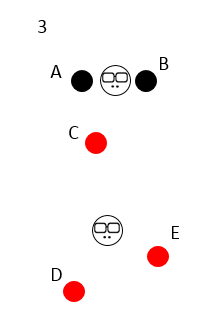然后开始换老大啦，2个初始中心点消失，重新在2个类分别中心的位置出现2个新的中心点，这2个新的中心点离类别里样本的距离之和必须是最小的；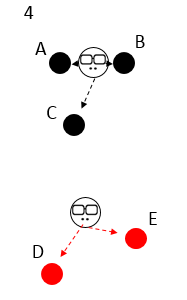新的老大出现，类别的划分也不一样啦，C开始叛变，皈依新老大，因为他离新老大更近一点；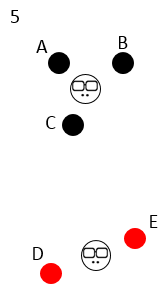新的老大消失，新新老大出现，发现划分的类别没有变化，帮派稳定，于是收敛。

``````import matplotlib.pyplot as plt
import random
import math
from copy import copy

#寻找新的中心点的函数
def new(group):
minimum=10000
o=[]
for x1 in range(min(group['x']),max(group['x'])):
for y1 in range(min(group['y']),max(group['y'])):
j=0
red_sum=0
while j<=len(group['x'])-1:
red_sum+=math.sqrt((group['x'][j]-x1)**2+(group['y'][j]-y1)**2)
j+=1
o.append(red_sum)
if(red_sum<minimum):
minimum=copy(red_sum)
x2=copy(x1)
y2=copy(y1)
return x2,y2

#根据中心点聚类并且着色的函数
def color(a,b,x,y):
i=0
red={'x':[],'y':[]}
blue={'x':[],'y':[]}
black={'x':[],'y':[]}
while i<=90:
distance0=math.sqrt((int(a[i])-x)**2+(int(b[i])-y)**2)
distance1=math.sqrt((int(a[i])-x)**2+(int(b[i])-y)**2)
distance2=math.sqrt((int(a[i])-x)**2+(int(b[i])-y)**2)
if (min(distance0,distance1,distance2)==distance0):
plt.plot(a[i],b[i],'ro',color='red')
red['x'].append(int(a[i]))
red['y'].append(int(b[i]))
elif (min(distance0,distance1,distance2)==distance1):
plt.plot(a[i],b[i],'ro',color='blue')
blue['x'].append(int(a[i]))
blue['y'].append(int(b[i]))
else:
plt.plot(a[i],b[i],'ro',color='black')
black['x'].append(int(a[i]))
black['y'].append(int(b[i]))
i+=1
return red,blue,black

def main():
#读取数据
file=open('d:/kmeans/data.txt')
a=[]
b=[]
data=line.strip().split(',')
a.append(data)
b.append(data)
#随机选取3个初始中心点
x=[random.randint(1,20),random.randint(1,20),random.randint(1,20)]
y=[random.randint(1,20),random.randint(1,20),random.randint(1,20)]
red,blue,black=color(a,b,x,y)
plt.plot(x,y,'x',color='red',markersize=15)
plt.plot(x,y,'x',color='blue',markersize=15)
plt.plot(x,y,'x',color='black',markersize=15)
plt.axis([0,25,0,25])
plt.show()
#循环执行函数，直到收敛
while (x,y!=new(red)) or (x,y!=new(blue)) or (x,y!=new(black)):
x,y=new(red)
x,y=new(blue)
x,y=new(black)
red,blue,black=color(a,b,x,y)
plt.plot(x,y,'x',color='red',markersize=15)
plt.plot(x,y,'x',color='blue',markersize=15)
plt.plot(x,y,'x',color='black',markersize=15)
plt.axis([0,25,0,25])
plt.show()
file.close()

if __name__=='__main__':
main()
``````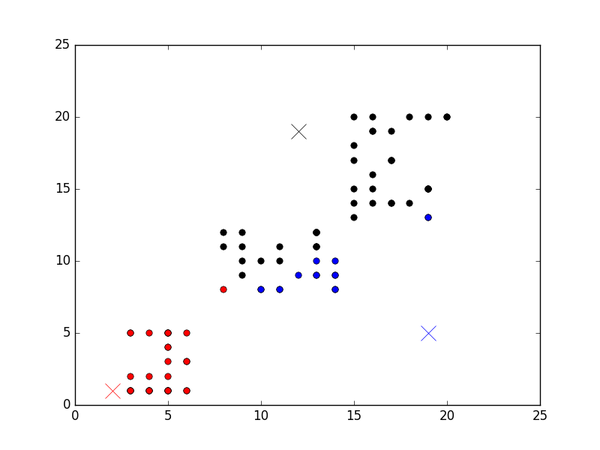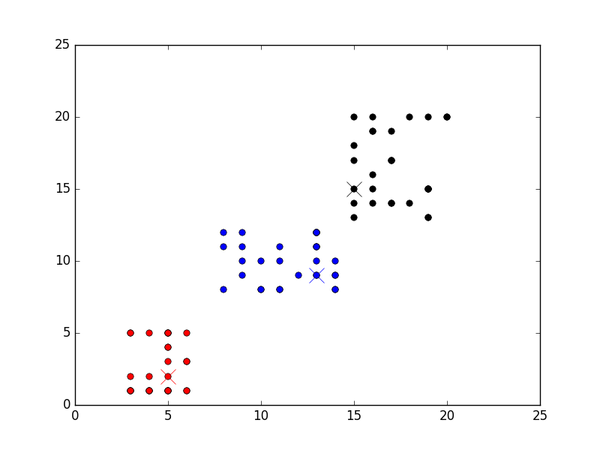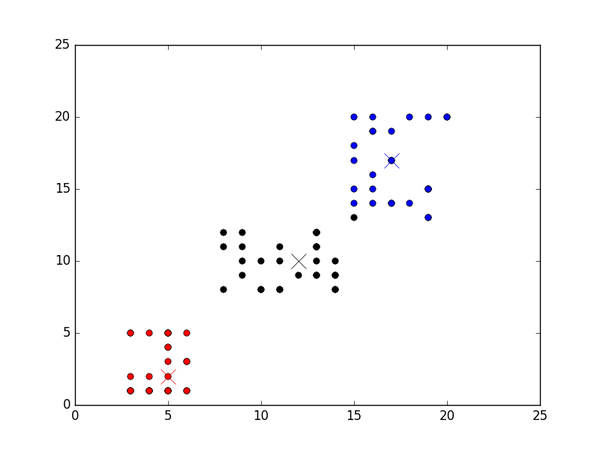【知乎日报】千万用户的选择，做朋友圈里的新鲜事分享大牛。